Next: CLP() Up: Programming with a Constraint Previous: Programming with a Constraint

## Primitive Constraints

The traditional model of a computer store admits only two possible states for a variable: assigned or unassigned. Constraint programming uses a generalisation of this model. A so-called constraint store can hold partial information about a variable, expressed as constraints on the variable. In this model, an unassigned variable is an unconstrained variable. An assigned variable is maximally constrained: no further non-redundant constraints can be imposed on the variable, without introducing an inconsistency.

Primitive constraints are the constraints that can be held in the constraint store. The simplest constraint store is the ordinary single-assignment store used in functional programming. In our terms it is a constraint store in which all constraints have the form Variable = Value.

The first generalisation is the introduction of the logical variable. This allows information of the form Variable = Term to be stored, where Term is any term in first-order logic. For example it is possible to store X = f(Y). The same representation can be used to store partial information about X. Thus if nothing is known about the argument of f we can store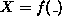. This is the model used in logic programming, and in particular by Prolog.

The storage model used by logic programming has a weakness, however. This is best illustrated by a simple example. The equation X-3 = Y+5 is rejected because logic programming does not associate any meaning with - or + in such an equation.

The extension of logic programming to store equations involving mathematical functions was an important breakthrough. Equations involving mathematical functions are passed to the constraint store, and checked by a specialised solver. In fact not only (linear) equations but also inequations can be checked for consistency by standard mathematical techniques. It is necessary, each time a new equation or inequation is encountered, to check it against the complete set of equations encoutered so far.

Linear equations and inequations are examples of primitive constraints. Thus we have an example of a constraint store. Further constraint stores can be built for different classes of primitive constraints, by designing constraint solvers specifically for those classes of constraints.

We use the term storage model, rather than data model, because the facility to store constraints is independent of the choice of data model - object-oriented, temporal etc. On the other hand the term storage model as used here does not refer to any physical representation of the stored information.

Definition A constraint store is a storage model which admits primitive constraints of a specific class. Each new primitive constraint that is added to the store is automatically checked for consistency with the currently stored constraints.

This definition of a constraint store specifies an equivalent operation to writing to a traditional store. However no equivalent to the read statement is specified. There are two important facilites useful for extracting information from a constraint store.

Firstly it is useful to retrieve all those constraints that involve a given variable, or set of variables. For example if the constraint store held three constraints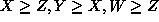the constraints involving X would be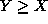and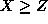. However retrieving only constraints explicitly involving a variable may not give a full picture of the entailed constraints on the variable. For example the store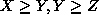entails. The mechanism necessary to return all the constraints and entailed constraints on a given variable or set of variables is termed projection. A very useful property of a class of primitive constraints is the property that the projection of a set of primitive constraints on a given variable, or set of variables, is also expressible as a set of primitive constraints. If the primitive constraints have this property it is possible, for example, to drop a variable from the constraint store when it is no longer relevant. This is the equivalent to reclaiming the store associated with a variable in a traditional programming language when the variable passes out of scope.

Secondly it is useful to retrieve particular kinds of entailed constraints from a constraint store. For example it is very useful to know when a constraint store entails that a particular variable has a fixed value. For example the constraint store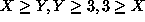, entails that both X and Y have the value 3.Next: CLP() Up: Programming with a Constraint Previous: Programming with a Constraint

Mark Wallace
Wed Sep 3 18:36:40 BST 1997